# Argumentation Logics Lecture 6 Argumentation with structured arguments

• Slides: 18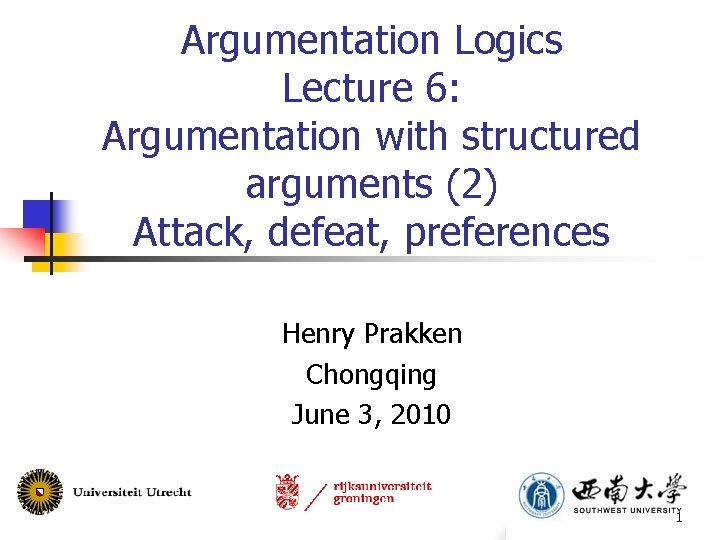Argumentation Logics Lecture 6: Argumentation with structured arguments (2) Attack, defeat, preferences Henry Prakken Chongqing June 3, 2010 1Overview n Argumentation with structured arguments: n n n Attack Defeat Preferences 2Argumentation systems n An argumentation system is a tuple AS = (L, -, R, ) where: n n n L is a logical language - is a contrariness function from L to 2 L R = Rs Rd is a set of strict and defeasible inference rules is a partial preorder on Rd If -( ) then: n n if -( ) then is a contrary of ; if -( ) then and are contradictories _ _ n = , = 3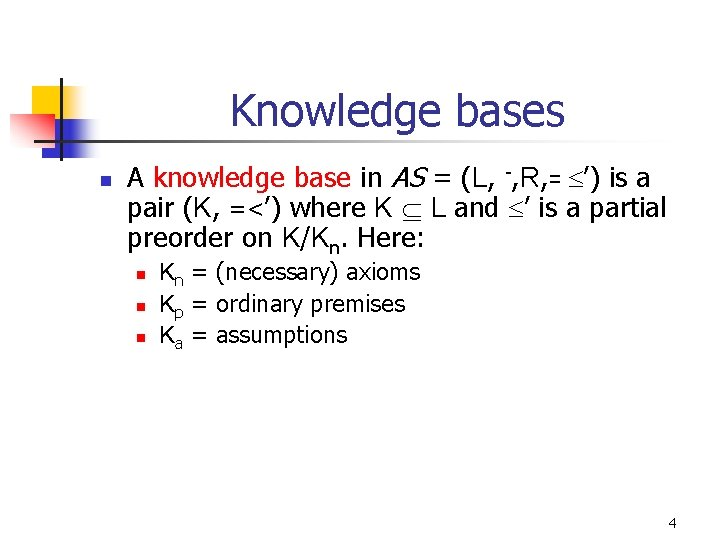Knowledge bases n A knowledge base in AS = (L, -, R, = ’) is a pair (K, =<’) where K L and ’ is a partial preorder on K/Kn. Here: n n n Kn = (necessary) axioms Kp = ordinary premises Ka = assumptions 4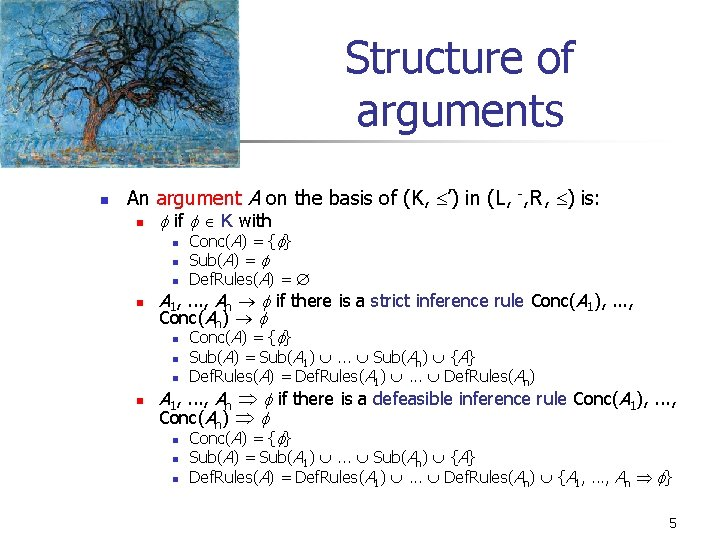Structure of arguments n An argument A on the basis of (K, ’) in (L, -, R, ) is: n if K with n n A 1, . . . , An if there is a strict inference rule Conc(A 1), . . . , Conc(An) n n Conc(A) = { } Sub(A) = Def. Rules(A) = Conc(A) = { } Sub(A) = Sub(A 1) . . . Sub(An) {A} Def. Rules(A) = Def. Rules(A 1) . . . Def. Rules(An) A 1, . . . , An if there is a defeasible inference rule Conc(A 1), . . . , Conc(An) n n n Conc(A) = { } Sub(A) = Sub(A 1) . . . Sub(An) {A} Def. Rules(A) = Def. Rules(A 1) . . . Def. Rules(An) {A 1, . . . , An } 5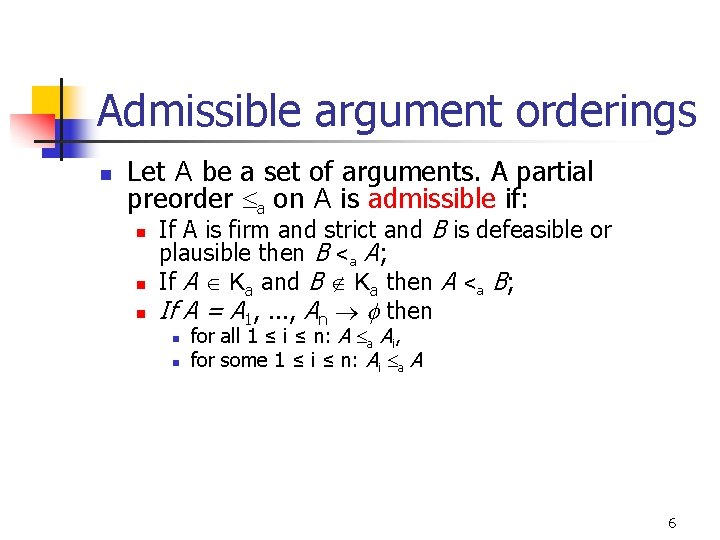Admissible argument orderings n Let A be a set of arguments. A partial preorder a on A is admissible if: n n n If A is firm and strict and B is defeasible or plausible then B <a A; If A Ka and B Ka then A <a B; If A = A 1, . . . , An then n n for all 1 ≤ i ≤ n: A a Ai, for some 1 ≤ i ≤ n: Ai a A 6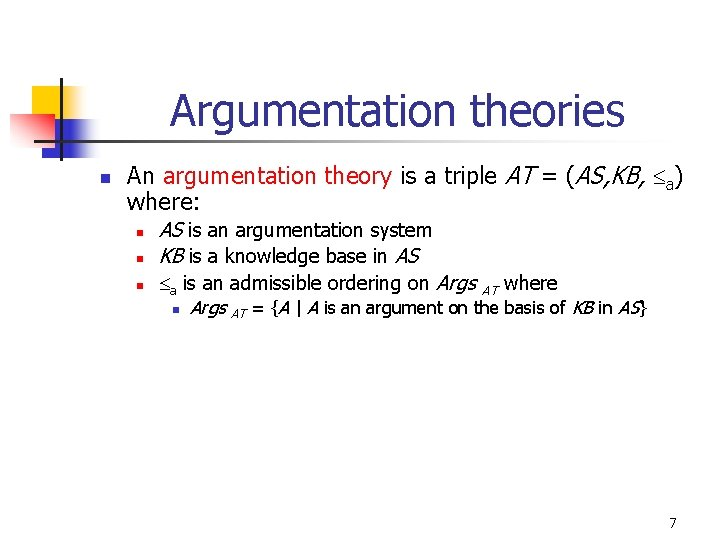Argumentation theories n An argumentation theory is a triple AT = (AS, KB, a) where: n n n AS is an argumentation system KB is a knowledge base in AS a is an admissible ordering on Args AT where n Args AT = {A | A is an argument on the basis of KB in AS} 7Attack and defeat (with - = ¬ and Ka = ) n A rebuts B (on B’ ) if n n n A undercuts B (on B’ ) if n n Conc(A) = ¬Conc(B’ ) for some B’ Sub(B ); and B’ applies a defeasible rule to derive Conc(B’ ) Naming convention implicit Conc(A) = ¬B’ for some B’ Sub(B ); and B’ applies a defeasible rule A undermines B if n Conc(A) = ¬ for some Prem(B )/Kn; A defeats B iff for some B’ n n n A rebuts B on B’ and not A <a B’ ; or A undermines B and not A <a B ; or A undercuts B on B’ 8Example cont’d R: n r 1: n r 2: n r 3: n r 4: n r 5: n r 6: n r 7: n r 8: p q p, q r s t t ¬r 1 u v v, q ¬t p, v ¬s s ¬p Kn = {p}, Kp = {s, u} 9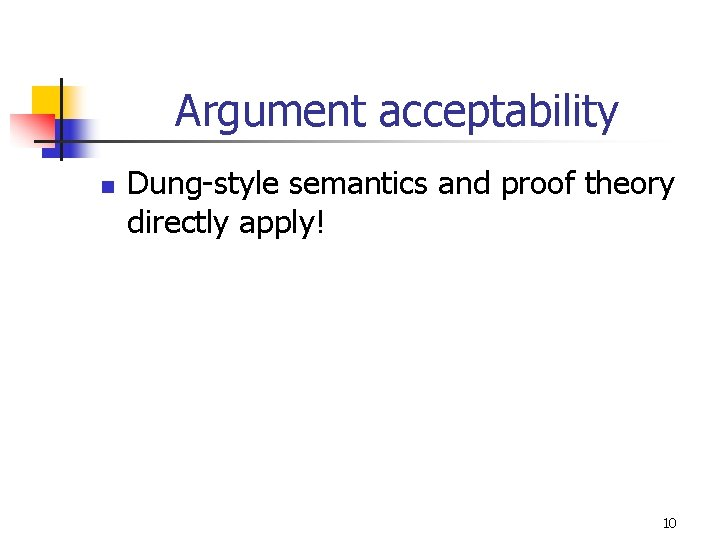Argument acceptability n Dung-style semantics and proof theory directly apply! 10The ultimate status of conclusions n With grounded semantics: n n With preferred semantics: n n A is justified if A g. e. A is overruled if A g. e. and A is defeated by g. e. A is defensible otherwise A is justified if A p. e for all p. e. A is defensible if A p. e. for some but not all p. e. A is overruled otherwise (? ) In all semantics: n n n is justified if is the conclusion of some justified argument (Alternative: if all extensions contain an argument for ) is defensible if is not justified and is the conclusion of some defensible argument is overruled if is not justified or defensible and there exists an overruled argument for 11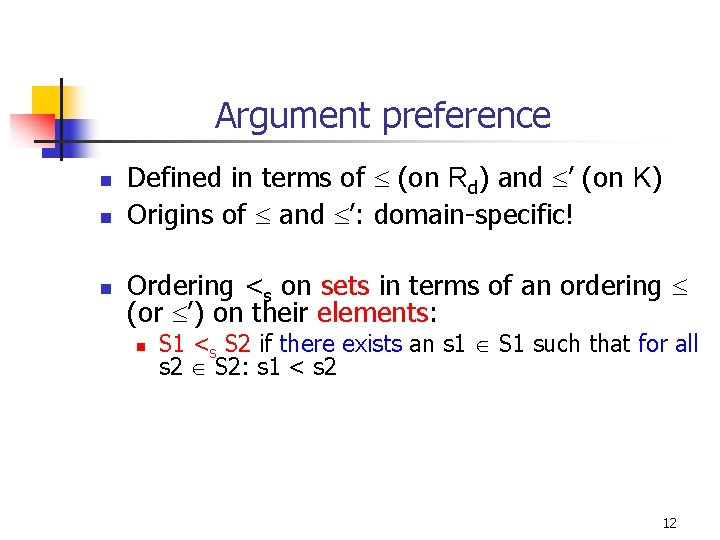Argument preference n n n Defined in terms of (on Rd) and ’ (on K) Origins of and ’: domain-specific! Ordering <s on sets in terms of an ordering (or ’) on their elements: n S 1 <s S 2 if there exists an s 1 S 1 such that for all s 2 S 2: s 1 < s 2 12Argument preference: some notation n An argument A is: n if K with n n n A 1, . . . , An if there is a strict inference rule Conc(A 1), . . . , Conc(An) n n n Def. Rules(A) = Last. Def. Rules(A) = Def. Rules(A 1) . . . Def. Rules(An) Last. Def. Rules(A) = Last. Def. Rules(A 1) . . . Last. Def. Rules(An) A 1, . . . , An if there is a defeasible inference rule Conc(A 1), . . . , Conc(An) n n Def. Rules(A) = Def. Rules(A 1) . . . Def. Rules(An) {A 1, . . . , An } Last. Def. Rules(A) = {A 1, . . . , An } 13Example Rd : n r 1: p q n r 2: p r n r 3: s t Rs: q, r ¬t K: n p, s 14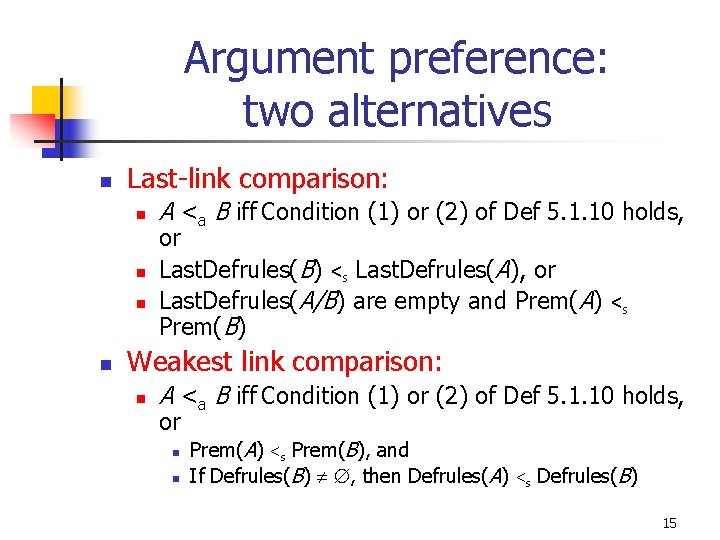Argument preference: two alternatives n Last-link comparison: n n A <a B iff Condition (1) or (2) of Def 5. 1. 10 holds, or Last. Defrules(B) <s Last. Defrules(A), or Last. Defrules(A/B) are empty and Prem(A) Prem(B) <s Weakest link comparison: n A <a B iff Condition (1) or (2) of Def 5. 1. 10 holds, or n n Prem(A) <s Prem(B), and If Defrules(B) , then Defrules(A) <s Defrules(B) 15Last link vs. weakest link (1) R: n r 1: p q n r 2: p, q r n r 3: s t n r 4: t ¬r 1 n r 5: u v n r 6: v ¬t r 3 < r 6, r 5 < r 3 K: n p, s, u 16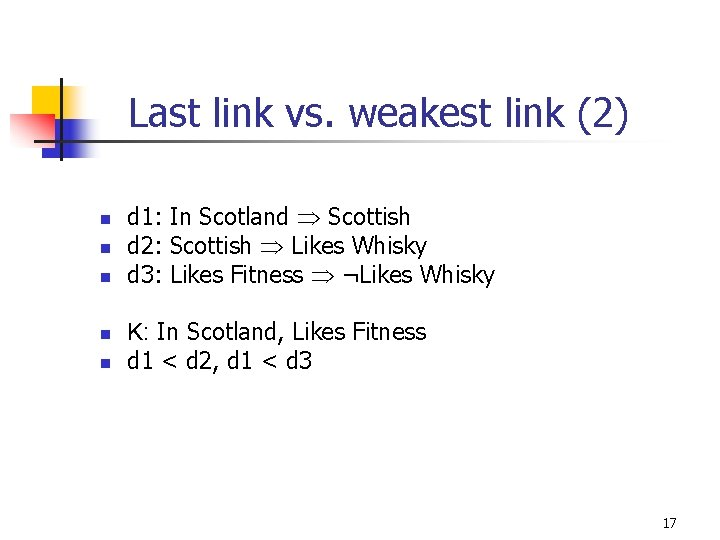Last link vs. weakest link (2) n n n d 1: In Scotland Scottish d 2: Scottish Likes Whisky d 3: Likes Fitness ¬Likes Whisky K: In Scotland, Likes Fitness d 1 < d 2, d 1 < d 3 17Last link vs. weakest link (3) n n n d 1: Snores Misbehaves d 2: Misbehaves May be removed d 3: Professor ¬May be removed K: Snores, Professor d 1 < d 2, d 1 < d 3 18